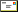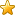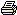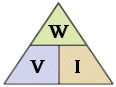Search the siteEmailBookmarkPrint

Wh ?

 What's the difference between Watts and Watt hours?

Sometimes there is a bit of confusion between the terms Watts and Watt hours. Fact is, Watts and Watt hours are very different beasts.

A Watt is a measure of Power. A Watt hour is a measure of Energy.

A Watt can be used to measure power in many different forms, for example we use Watts to measure electrical power, and we use also use Watts to measure mechanical power ( you car engine's power is can be measured in kiloWatts ( 1000 Watts ). To keep it simple, we'll stick with electrical power for this article.

We can work out watts using this simple formula.Where : W = Watts V = Volts I = Amps Watts = Volts X Amps Volts = Watts / Amps Amps = Watts / Volts

If we have a 21 Watt automotive light bulb, like a typical brake light, and we know it runs on 12V, then using the formula above we can work out it will draw 1.75 amps. The Watts is how much power the bulb is using.

A automotive spot light might be rated at 100 watts, so at 12 volts, it will draw 8.3 amps.

We can also use Volts and Amps to work out how many watts is used in a circuit. If we feed a heater circuit, like a bread toaster, with 240 volts, and its drawing 4 amps, then we can work out its drawing 960 watts.

So what's a Watt hour?

Watt hours is a measure of Energy. Energy is Power by Time.

A 100 Watt light bulb can use 100 Watts for 1 second, 1 hour, 1 decade. Its still a 100 Watt light bulb, but we need more energy to power the bulb for 1 hour than we need for 1 second, and a lot more for 1 decade. We call this measure of power over time a Watt hour ( Wh ).

If I power my 100 Watt light bulb for 1 hour, its easy to work out, we used 100 Wh's of energy. Watt hours = Watts by hours

If I power my 100 watt light bulb for 30 minutes, then we used 50 Wh's of energy ( 100W X 0.5h = 50Wh ). We used half as much energy as we needed to power the same bulb for one hour. If I power the same bulb to 2 hours, then we used 200Wh.

This is why our electricity bills measured in Watt hours, its a true measure of how much energy we used. Sometimes a house is using very little power, other times its using much more. The Watt hour is a measure of the total energy used.

We can also use Wh to measure the energy going into a battery from a power source, like a solar panel or wind turbine. If I have a 50 watt solar panel, and it was exposed to full sun for 5 hours, then it made 250Wh.

Now the fun bit. We can take the power out at a different rate than we put it in. If my 50 watt solar panel produced 50 watts for 5 hours, 250Wh, and this power was stored in a battery, we can take the same energy back out of the battery at a different rate. If I connect up a 100 watt light bulb, it will drain that 250Wh in 2.5 hours. If we used a 5 watt light bulb, it would last 50 hours. The same energy is used in every case.

In reality, its not a perfect world and there are losses every time energy is transformed from one type to another ( solar to electricity to chemical to electricity to heat and light ), so we will ALWAYS get less out than we put in.

You may hear the wind turbine builders comment that its better to build a turbine that can use low winds than high winds. A wind turbine that makes 100 watts for 10 hours will give you twice the energy as a turbine that makes 500 watts for one hour. In reality, the maximum power a wind turbine can make means very little when it comes to the energy it can supply. And as most wind is slow wind, you are better building a turbine that can make the most power in low winds, even if it means it cant make a lot of power in high winds.

Its like a big V8 sports car and a small economical 4 cylinder hatch. The V8 can go faster, and has much more power, but if you give both the V8 and the 4 cylinder the same amount of petrol ( energy ), its the 4 cylinder that will go much further.